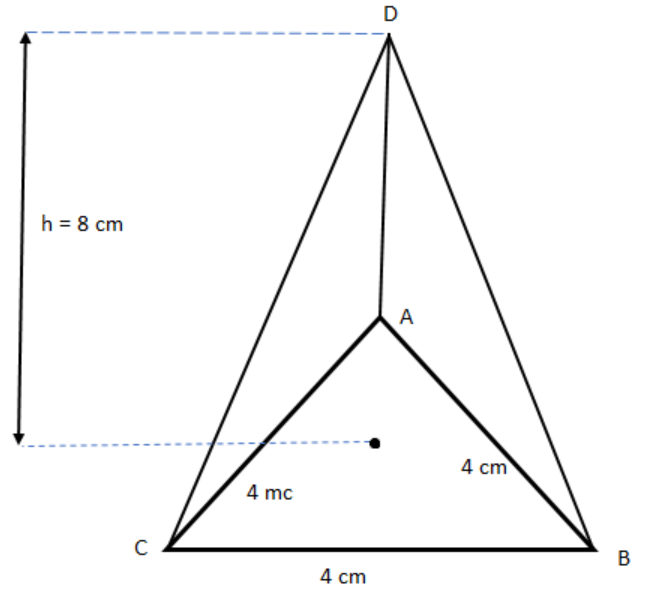Find the lateral surface area and total surface area of an equilateral triangle-based prism with height 8 cm and base 4 cm.Verified
147.3k+ views
Hint: First of all, draw the diagram of the equilateral triangle-based prism to have a clear idea of what we have to find. Then use the formula for finding L.S.A and T.S.A of the equilateral triangle-based prism.

Complete step-by-step solution:
Given that the base of the prism is an equilateral triangle with side 4 cm as shown in the given figure:Let $p$ be the perimeter of the base of the prism i.e., the perimeter of equilateral $\Delta ABC$.
So, $p = 4 + 4 + 4 = 12{\text{ cm}}$
Given height of the prism $h = 8\;{\text{cm}}$
We know that the lateral surface area of the triangle-based prism is given by $p \times h$ where $p$ is the perimeter of the base triangle and $h$ is the height of the prism.
Therefore, L.S.A of the prism $= p \times h = 12 \times 8 = 96{\text{ c}}{{\text{m}}^2}$
Now, consider the area of equilateral $\Delta ABC$.
We know that the area of the equilateral triangle with side $a$ is given by $\dfrac{{{a^2}}}{4}$.
So, area of $\Delta ABC = \dfrac{{\sqrt 3 {{\left( 4 \right)}^2}}}{4} = 4\sqrt 3$
We know that the total surface area of the triangle based prism with base area $A$, perimeter $p$ and $h$ is the height of the prism is given by $2 \times A + p \times h$.
Therefore, the total surface area of the prism $= 2 \times 4\sqrt 3 + 12 \times 8$
$= 8\sqrt 3 + 96 \\ = 8\left( {1.73} \right) + 96 \\ = 13.84 + 96 \\ = 109.84{\text{ c}}{{\text{m}}^2}$
Thus, the L.S.A of the prism is $96{\text{ c}}{{\text{m}}^2}$ and the T.S.A of the prism is ${\text{109}}{\text{.84 c}}{{\text{m}}^2}$.

Note: The lateral surface area of the triangle-based prism is given by $p \times h$ where $p$ is the perimeter of the base triangle and $h$ is the height of the prism. The total surface area of the triangle based prism with base area $A$, perimeter $p$ and $h$ is the height of the prism is given by $2 \times A + p \times h$.